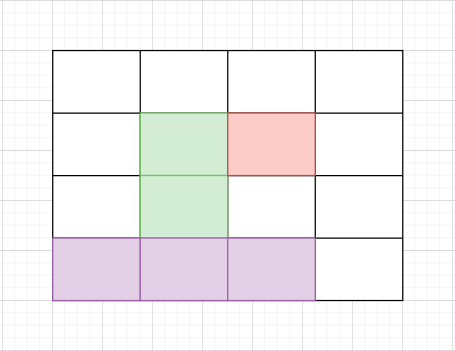# Maximum Size Rectangle Sub-matrix With All 1's

Posted: 20 Nov, 2020
Difficulty: Hard

## PROBLEM STATEMENT

#### You are given an 'N' * 'M' sized binary-valued matrix 'MAT, where 'N' is the number of rows and 'M' is the number of columns. You need to return the maximum size (area) of the submatrix which consists of all 1’s i.e. the maximum area of a submatrix in which each cell has only the value ‘1’.``````In the above image, areas in green, red, and violet color are all submatrices of the original 4x4 matrix.
``````

#### Note:

``````1. Binary valued matrix has only two values in each cell : 0 and 1.
2. A submatrix is a matrix formed by selecting certain rows and columns from a larger matrix.
3. The area of a matrix with 'h' rows and 'w' columns is equal to 'h' * 'w'.
``````
##### Input Format:
``````The first line of the input contains an integer 'T' denoting the number of test cases.

The first line of each test case contains two space-separated integers 'N' and 'M', where 'N' = the number of rows in the given matrix and 'M' = the number of columns in the given matrix.

Then 'N' lines follow for each test case. Each line contains 'M' space-separated integers (either 1 or 0) denoting matrix elements.
``````
##### Output Format:
``````For each test case print in a single line the area of maximum size submatrix of all 1’s in the given matrix on a new line.

The output of each test case will be printed in a separate line.
``````
##### Note:
``````You do not need to print anything, it has already been taken care of. Just implement the given function.
``````
##### Constraints:
``````1 <= 'T' <= 50
1 <= 'N', 'M' <= 100

Time Limit: 1 sec
``````Approach 1
1. We start from the first row and move downwards.
2. We create three 1-dimensional arrays HEIGHT[], LEFT[], RIGHT[].
3. ‘HEIGHT’[i]: stores the number of current continuous 1’s in column i.
4. LEFT[i] : stores the leftmost index ‘j’ such that all indices say ‘K’, ‘K’ belongs to [j, i], ‘HEIGHT’[k] >= ‘HEIGHT’[i].
5. RIGHT [i]: stores the rightmost index ‘j’ such that all indices say ‘K’, ‘K’ belongs to [i, j], ‘HEIGHT’[k] >= ‘HEIGHT’[i].
6. By the above definitions, it’s easier to figure out that we can update our maximum area with the value (‘HEIGHT’[i] * (RIGHT [i] - LEFT [i])).
7. Now let’s think how will we update the above three 1-dimensional arrays itself.
8. Initialize the ‘HEIGHT’[] array with 0.
9. Iterate through the rows from 0 to N-1.
10. For each column:
11. If the current value (MAT [i][j]) is 1, then update ‘HEIGHT’[j]++.
12. Else reset the value of ‘HEIGHT’[j] to 0.
13. Note that:
14. Initially, ‘HEIGHT’ was initialized to 0. So LEFT[] array should be initialised to 0 as per definition.
15. And similarly, RIGHT[] array should be initialized to M.
16. Updating the LEFT[] array in each iteration among rows 0 to N-1:
17. We scan from left to right.
18. Initialize LEFTBOUNDARY = 0, which means left boundary for all 1’s in the current row.
19. Iterate in the current row:
20. If the current value  (MAT [i][j]) is 1, then you can reset the LEFT[j] from 0 to LEFTBOUNDARY.
21. Else left[j] = 0 and update LEFTBOUNDARY to j+1. As the current value is 0, so next LEFTBOUNDARY for all the cells in the Matrix ahead of column j is at least j+1.
22. Similarly, for the RIGHT[] array  in each iteration among rows 0 to N-1:
23. We scan from right to left.
24. Initialize RIGHTBOUNDARY = 0, which means right boundary for all 1’s in the current row.
25. Iterate in the current row:
26. If the current value  (MAT[i][j]) is 1, then you can reset the RIGHT[j] from M to RIGHTBOUNDARY.
27. Else RIGHT[j] = 0 and update RIGHTBOUNDARY to j-1. As current value is 0, so next RIGHTBOUNDARY for all the cells in the Matrix before column j is atmost j-1.